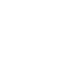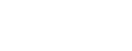# Chemistry Paper 1 Questions and Answers - Form 3 End Term 1 Exams

• Answer all the questions in the spaces provided.
1. Dyes W,X, Y and Z were analysed using P as the purest sample of the dye required by a chemist .the result obtained were as shown on the chromatogram below.1. What names are given to the lines marked?
1. A 1mk
2. B 1mk
2. State with a reason the most unsuitable dye for the chemist to use . 2mks
2.
1. Distinguish between allotropes and isotopes. 2mks
2. Name the two allotropes of sulphur. 2mks
3. The table below shows the atomic numbers of elements F G and H.
 Element F G H Atomic number 4 12 20
Which of the elements is the least reactive? Explain 2mks
4. Classify the changes below as physical or chemical changes.
 Type of change Magnetization of iron Heating iron until it softens Souring of milk
5. A student prepared ammonia gas and wanted to make a solution in water. Complete the diagram to show how he made the solution.6. Element J belong to period 3 and group V.
1. Write the electron arrangement of J. 1mk
2. The equation of the reaction when J reacts with oxygen gas. 2mks
7. A fixed mass of a gas has a volume of 200cm3 at a temperature of 47°C and 750mmHg. Calculate the volume the gas would occupy at 7°C and 750mmHg pressure. 3mks
8. The diagram below represents a luminous flame.Mark and label on the diagram the hottest and coolest parts 2mks
9. Burning magnesium was lowered in a gas jar full of dry sulphur IV oxide.
1. State the observation that was made. 2mks
2. Write the equation for the reaction occurring. 1mk
3. State the reducing agent in the above reaction. 1mk
10. Draw a dot (.) and cross (X) diagram to show the bonding in the molecules of :
(Atomic number of carbon is 6, Oxygen is 8 and hydrogen is 1)
1. Carbon II oxide.
2. Water.
11. Explain the following observations
1. Magnesium is a better electrical conductor than sodium. 1mk
2. A solution of aluminum chloride has PH of 3.0. 1mk
12. A compound W consist of 26.7%carbon, 2.2% hydrogen and the rest oxygen. It has a relative formula Mass of 90(C=12, H=1, O=16,)
1. Determine it empirical formula. 2mks
2. Workout its molecular formula 2mks
13. Hydrogen was passed over copper II oxide in a combustion tube.
1. Write an equation for the reaction that took place. 1mk
2. What observation was made in the combustion tube? 2mks
3. Name two other gases which could be used to reduce copper II oxide. 2mks
14.
1. State grahams law of diffusion. 1mk
2. 20cm3 of oxygen diffused through a porous partition in 70s .how long would it take 20cm3. Of sulphur VI oxide to diffuse through the same portion under the same condition. 3mks
15. The set up below was used to collect gas Z produced by the reaction between water and calcium metal.1. Give a chemical test for gas Z. 2mks
2. Write the equation of the reaction between the calcium metal and water. 2mks
16. The diagram below shoes the bonding between aluminium chloride and ammonia1. Name the type of bond labeled
1. X. 1mk
2. Y 1mk
2. How many electrons are used for bonding in the molecule? 1mk
17. Graphite is a non metal, yet it conduct an electric current .explain this observation. 1mk
18. A sample of air contaminated with carbon monoxide and sulphur dioxide was passed through the apparatus shown in the diagram below.Which contaminant was removed by passing the contaminated air through the apparatus?
Explain. (2mks)
19. Explain how you would show chemically that a given gas is ammonia. 2mks
20. Chlorine water was exposed to sunlight for a few hours .Colourless gas F was collected in the boiling tube as shown.1. Name gas F. 1mk
2. Write an equation for the formation of gas F.2mks
21. The equation below shows the action of heat on three metal nitrates.
2S(NO3)2 → 2SO + 4NO2 +O2
2TNO3  →  2TNO2 + O2
2UNO3 → 2U +2NO2 + O2
1. Arrange the metals S,T and U in the order of reactivity from least to the most reactive. 3mks
2. Give one element that could be used. 1mk
22.
1. Define the term transition temperature concerning the allotropes of sulphur. 1mk
2. Draw the structure of a sulphur molecule. 1mk
23. Dilute hydrochloric acid was added to a compound Z of sodium. The solid reacted with the acid to form a colorless solution H and a colourless gas K which formed a white precipitate when bubbled through lime water.
Write the formula of:
1.
1. Compound Z 1mk
2. Colorless gas K 1mk
2. Write an equation for the reaction that took place. 2mks
24. The diagram below represents the extraction of sulphur by Frasch process1. Name the substance that passes through tube;
I  ½ mk
II  ½ mk
2. What is the purpose of hot compressed air in this process? (1mk)
25. A student used the setup below to prepare oxygen gas in the laboratory. Study it and answer the questions that follow.1. Identify solid K. 1mk
2. Name the apparatus labeled L. 1mk
3. Write an equation for the reaction in the conical flask. 2mk
26. Nitrogen gas is used as an inert atmosphere in electric lamps.
1. Explain in terms of structure and bonding why nitrogen gas is so stable .2mks
2. Give a reason why despite this stability nitrogen reacts with burning magnesium metal. 1mk## Marking Scheme

1.
1.
1. A-Solvent front ✓1
2. B-Baseline  ✓1
2.
• Z ✓1
Z is the most impure woth 3 substances not in the pure dye ✓1
2.
1.
• Allotropes are two or more forms of an element in the same physical state. ✓1
• Isotopes are atoms of the same element having the same atomic number but different mass numbers. ✓1
2.
• Rhombic Sulphur ✓1
• Monoclinic sulphur ✓1
3.
• F ✓1
F has the smallest atomic radius/ size and has the least tendency to lose electrons ✓1
4.
 Type of change Magnetization of iron Physical change ✓1 Heating iron until it softens physical change ✓1 Souring of milk Chemical change ✓1
5.6.
1. 2.8.5 ✓1
2. 4J(s) + 3O2(g) → 2J2O3(g)  ✓2
OR
4J(s) + 5O2(g) → 2J2O5(g)
Award 2 marks if correct state symmbols are there
7. V= 200cm3                                V2 = ?
T1 = 47°C = 47+273= 320K ✓½   T2 = 7ºC = 7+273 =280K  ✓½
P1 =750mmHg                              P2 =750mmHg= 200 × 280 ✓1
320
=175cm3  ✓1
8.9.
1. White ash ✓1 and yellow specks formed✓1.
2. 2Mg(s) + SO2(g) → 2MgO(s) + S(s) ✓1
3. Magnesium  ✓1
10.
1.2.11.
1. Magnesium has 2 ✓½ delocalised electrons compared to sodium which has 1✓½.
2. Aluminium chloride hydrolyses in solution to form an acidic solution.✓1
12.
1. %oxygen =100 − (26.7 + 2.20)=71.1% ✓½
C      H       O
%comp. W  26.7  2.2   71.1
R.A.M           12     1      16
No. of moles 26.7  2.2   71.1
12     1      16
2.2    2.2     4.4  ✓½
2.2    2.2     4.4
2.1    2.2     2.2
1      1       2
E. F is C1H1O2 = CHO2  ✓1
2. (CHO2)n = 90  ✓½
12n + n + 32n = 90
45n = 90
n=2 ✓½
M.F is C2H2O4  ✓1
13.
1. H2(g) +CuO(s) → H2O(l) + Cu(s) ✓1
2. Black ✓1 Copper (II) Oxide turned brown ✓1
3. Ammonia gas ✓1 and carbon (II) oxide gas ✓1
14.
1. Graham's law of diffusion states that under the same conditions of temperature and pressure the rate of diffusion of a gas is inversely proportional to the square root of its density.
2. Mo2 = 2 × 16 =32 ✓½ Mso3 =(32+16×3) = 80 ✓½
To2 =70secs                Tso3 =?

3.Tso3√80
70      √32
Tso3 = 70√80 = 70×8.944 ✓1 =110secs ✓1
√32          5.657
15.
1. It burns with a pop sound. ✓1
2. Ca(s) + 2H2O(l) → Ca(OH)2(aq) + H2(g) ✓2
16.
1.
1. X- co-ordiante/ dative bond ✓1
2. Y-covalent bond ✓1
2. 14 electrons ✓1
17. Graphite has delocalised electrons in its structure.✓1
18.
• Sulphur(IV)Oxide ✓1
Carbon(II)Oxide gas does not react with lime water ✓1 nor Conc. H2SO4 but SO2 being acidic is absorbed by the lime water.✓1
19. Dip a glass rod in ✓1 concentrated hydrochhloric acid and introduce it near the unknown gas. If dense ✓1 white fumes are formed, them the gas is ammonia
OR
If it turns dump red ✓1 litmus paper blue ✓1, It is ammonia.
20.
1. Gas F- Oxygen gas ✓1
2. 2HOCl(aq) → 2HCl(aq) + O2(g) ✓2
21.
1. U -least reactive
S
T-most reactive ✓3
2. silver or mercury ✓1
22.
1. Transition temperature is the temperature at which one allotrope of Sulphur changes to another. ✓1

2.✓1
23.
1.
1. Na2CO3 ✓1
2. CO2 ✓1
2. Na2CO3(s) + 2HCl(aq) → CaCl2(aq) + H2O(l) +CO2(g) ✓2
24.
1.
I - Molten sulphur ✓1
II - Superheated water ✓1
2. To force out more molten Sulphur from underground. ✓1
25.
1. Solid K - Sodium peroxide ✓1
2. Beehive stand ✓1
3. 2Na2O2(s) + 2H2O(l) → 4NaOH(aq) + O2(g) ✓2
26.
1. The atoms of Nitrogen are bonded by three strong covalent bonds ✓1 which need a lot of energy to break. ✓1
2. Burning Magnesium releases a lot of heat energy which gets absorbed by the Nitrogen molecule breaking the tripple covalent bond. ✓1

• ✔ To read offline at any time.
• ✔ To Print at your convenience
• ✔ Share Easily with Friends / Students